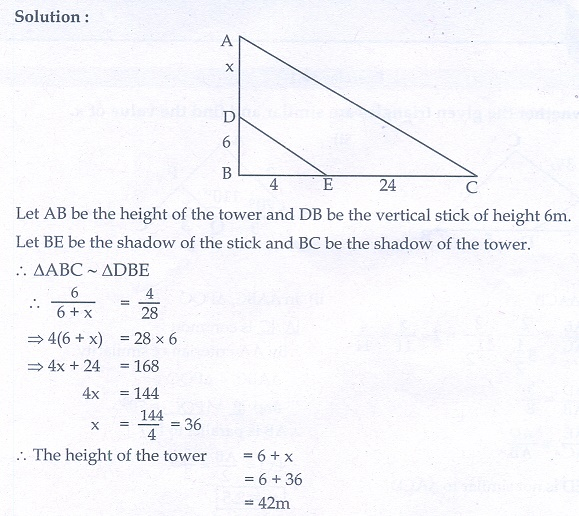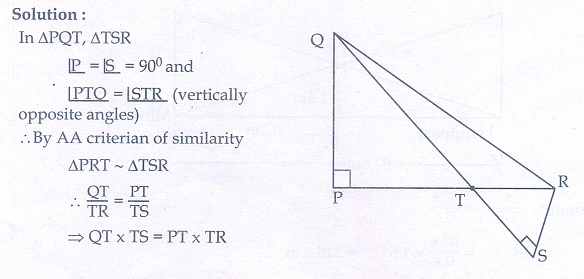Home | | Maths 10th Std | Exercise 4.1: Similarity and Similar triangles

# Exercise 4.1: Similarity and Similar triangles

Maths Book back answers and solution for Exercise questions - Mathematics : Geometry: Similarity and Similar triangles: Exercise Problem Questions with Answer

Exercise 4.1

1. Check whether the which triangles are similar and find the value of x.2. A girl looks the reflection of the top of the lamp post on the mirror which is 66 m away from the foot of the lamppost. The girl whose height is 12.5 m is standing 2.5  m away from the mirror. Assuming the mirror is placed on the ground facing the sky and the girl, mirror and the lamppost are in a same line, find the height of the lamp post.3. A vertical stick of length 6 m casts a shadow 400 cm long on the ground and at the same time a tower casts a shadow 28 m long. Using similarity, find the height of the tower.4. Two triangles QPR and QSR, right angled at P and S respectively are drawn on the same base QR and on the same side of QR. If PR and SQintersect at T, prove that PT × TR = ST × TQ.5. In the adjacent figure, ΔABC is right angled at C and DE ┴ AB . Prove that  ΔABC ~ ΔADE and hence find the lengths of AE and DE.6. In the adjacent figure, ΔACB ~ ΔAPQ . If BC = 8 cm, PQ = 4 cm, BA = 6.5 cm and AP = 2.8 cm, find CA and AQ7. If figure OPRQ is a square and MLN = 90° . Prove that

(i) ΔLOP ~ ΔQMO

(ii) ΔLOP ~ ΔRPN

(iii) ΔQMO ~ ΔRPN

(iv) QR2  = MQ ×RN8. If ΔABC ~ ΔDEF such that area of ΔABC is 9cm2 and the area of ΔDEF is 16cm2 and BC = 2.1 cm. Find the length of EF9. Two vertical poles of heights 6 m and 3 m are erected above a horizontal ground AC. Find the value of y10. Construct a triangle similar to a given triangle PQR with its sides equal to 2/3 of the corresponding sides of the triangle PQR (scale factor 2/3).11. Construct a triangle similar to a given triangle LMN with its sides equal to 4/5 of the corresponding sides of the triangle LMN (scale factor 4/5).12. Construct a triangle similar to a given triangle ABC with its sides equal to 6/5 of the corresponding sides of the triangle ABC (scale factor 6/4).13. Construct a triangle similar to a given triangle PQR with its sides equal to 7/3 of the corresponding sides of the triangle PQR (scale factor 7/3).1.(i) Not similar (ii) Similar,2.5

2. 330 m

3. 42 m

5. 15/13, 36/13

6. 5.6 cm, 3.25 cm

7. 2.8 cm

9. 2 m

Tags : Problem Questions with Answer, Solution | Mathematics , 10th Mathematics : UNIT 4 : Geometry
Study Material, Lecturing Notes, Assignment, Reference, Wiki description explanation, brief detail
10th Mathematics : UNIT 4 : Geometry : Exercise 4.1: Similarity and Similar triangles | Problem Questions with Answer, Solution | Mathematics